# Maharashtra Board Class 9 Maths Solutions Chapter 5 Linear Equations in Two Variables Problem Set 5

## Maharashtra Board Class 9 Maths Solutions Chapter 5 Linear Equations in Two Variables Problem Set 5

Question 1.
Choose the correct alternative answers for the following questions.

i. If 3x + 5y = 9 and 5x + 3y = 7, then what is the value of x + y ?
(A) 2
(B) 16
(C) 9
(D) 7
(A) 2

ii. ‘When 5 is subtracted from length and breadth of the rectangle, the perimeter becomes 26’. What is the mathematical form of the statement ?
(A) x – y = 8
(B) x + y = 8
(C) x + y = 23
(D) 2x + y = 21
(C) x + y = 23

iii. Ajay is younger than Vijay by 5 years. Sum of their ages is 25 years. What is Ajay’s age?
(A) 20 years
(B) 15 years
(C) 10 years
(D) 5 years
(C) 10 years

Hints:
3x+ 5y = 9
5x + 3y = 7
8x + 8y = 16
∴ x + y = 2 .. [Dividing both sides by 8]

ii. Let the length of the rectangle be ‘x’ and that of breadth be ‘y’.
Perimeter of rectangle = 2[(x – 5) + (y – 5)]
∴ 26 = 2(x + y – 10)
∴ x + y – 10 = 13
∴ x + y = 23

iii. Let the age of Ajay bex years.
∴ x + (x + 5) = 25
∴ 2x = 20
∴ x = 10 years

Question 2.
Solve the following simultaneous equations.
i. 2x + y = 5 ; 3x – y = 5
ii. x – 2y = -1 ; 2x – y = 7
iii. x + y = 11 ; 2x – 3y = 7
iv. 2x + y = -2 ; 3x – y = 7
V. 2x – y = 5 ; 3x + 2y = 11
vi. x – 2y – 2 ; x + 2y = 10
Solution:
ii. 2x + y = 5 …(i)
3x – y = 5 …(ii)
2x + y = 5
+ 3x – y = 5
5x = 10
∴ x = 10/5
∴ x = 2
Substituting x = 2 in equation (i),
2(2) + y = 5
4 + y = 5
∴ y = 5 – 4 = 1
∴ (2, 1) is the solution of the given equations.

ii. x – 2y = -1
∴x = 2y – 1 … .(i)
∴ 2x – y = 7 ….(ii)
Substituting x = 2y – 1 in equation (ii),
2(2y – 1) – y = 7
∴ 4y – 2 – y = 7
∴ 3y = 7 + 2
∴ 3y = 9
∴ y = 9/3
∴ y = 3
Substituting y = 3 in equation (i),
x = 2y – 1
∴ x = 2(3) – 1
∴ x = 6 – 1 = 5
∴ (5, 3) is the solution of the given equations.

iii. x + y = 11
∴ x = 11 – y …(i)
2x – 3y = 7 …….(ii)
Substituting x = 11 -y in equation (ii),
2(11 – y) – 3y = 7
∴ 22 – 2y – 3y = 1
∴ 22 – 5y = 7
∴ 22 – 7 = 5y
∴ 15 = 5y
∴ y = $$\frac { 15 }{ 5 }$$
∴ y = 3
Substituting y = 3 in equation (i),
x = 11 – y
∴ x = 11 – 3 = 8
∴ (8, 3) is the solution of the given equations.

iv. 2x + y = -2 …(i)
3x – y = 7 …(ii)
2x + y = -2
+ 3x – y = l
5x = 5
∴ x = $$\frac { 5 }{ 5 }$$
∴ x = 1
Substituting x = 1 in equation (i),
2x + y = -2
∴ 2(1) +y = -2
2 + y = -2
∴ y = – 2 – 2
∴ y = -4
∴ (1, -4) is the solution of the given equations.

v. 2x – y = 5
∴ -y = 5 – 2x
∴ y = 2x – 5 …(i)
3x + 2y = 11 ……(ii)
Substituting y = 2x – 5 in equation (ii),
3x + 2(2x – 5) = 11
∴ 3x + 4x- 10= 11
∴ 7x = 11 + 10
∴ 7x = 21
∴ x = $$\frac { 21 }{ 7 }$$
∴ x = 3
Substituting x = 3 in equation (i),
y = 2x – 5
∴ y = 2(3) – 5
∴ y = 6 – 5 = 1
∴(3,1) is the solution of the given equations.

vi. x – 2y = -2
∴ x = 2y – 2 …(i)
x + 2y = 10 …..(ii)
Substituting x = 2y – 2 in equation (ii),
2y – 2 + 2y = 10
∴ 4y = 10 + 2
∴ 4y= 12
∴ y = $$\frac { 12 }{ 7 }$$
∴ y = 3
Substituting y = 3 in equation (i),
x = 2y – 2
∴ x = 2(3) – 2
∴ x = 6 – 2 = 4
∴ (4, 3) is the solution of the given equations.

Question 3.
By equating coefficients of variables, solve the following equations. [3 Marks each]
i. 3x – 4y = 7 ; 5x + 2y = 3
ii. 5x + ly= 17 ; 3x – 2y = 4
iii. x – 2y = -10 ; 3x – 3y = -12
iv. 4x+y = 34 ; x + 4y = 16
Solution:
i. 3x – 4y = 7 …(i)
5x + 2y = 3 ….(ii)
Multiplying equation (ii) by 2,
10x + 4y = 6 …(iii)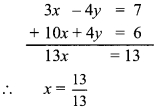∴ x = 1
Substituting x = 1 in equation (i),
3x – 4y = 7
∴ 3(1) – 4y = 7
∴ 3 – 4y = 7
∴ 3 – 7 = 4y
∴ -4 = 4y
∴ y = $$\frac { -4 }{ 4 }$$
∴ y = -1
∴ (1, -1) is the solution of the given equations.

ii. 5x + 7y = 17 …(i)
3x – 2y = 4 ….(ii)
Multiplying equation (i) by 2,
10x + 14y = 34 …(iii)
Multiplying equation (ii) by 7,
21x – 14y = 28 …..(iv)∴ x = 2
Substituting x = 2 in equation (ii),
3x – 2y = 4
∴ 3(2) – 2y = 4
∴ 6 – 2y = 4
∴ 6 – 4 = 2y
∴ 2 = 2y
∴ y = $$\frac { 2 }{ 2 }$$
∴ y = 1
∴ (2,1) is the solution of the given equations.

iii. x – 2y = -10 ….(i)
3x – 5y = -12 …….(ii)
Multiplying equation (i) by 3,
3x – 6y = -30 …(iii)
Subtracting equation (ii) from (iii),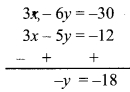∴ y = 18
Substituting y = 18 in equation (i),
x – 2y = -10
∴ x – 2(18) = -10
∴ x – 36 = -10
∴ x = -10 + 36 = 26
∴ (26, 18) is the solution of the given equations.

iv. 4x + y = 34 …(i)
x + 4y = 16 …… (ii)
Multiplying equation (i) by 4,
16x + 4y = 136 …(iii)
Subtracting equation (ii) from (iii),x = 8
Substituting x = 8 in equation (i),
4x + y = 34
∴ 4(8) + y = 34
∴ 32 + y = 34
∴ y = 34 – 32 = 2
∴ (8, 2) is the solution of the given equations.

Question 4.
Solve the following simultaneous equations.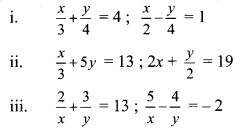Solution:
i. $$\frac{x}{3}+\frac{y}{4}=4$$
Multiplying both sides by 12,
4x + 3y = 48 …(i)
$$\frac{x}{2}-\frac{y}{4}=1$$
Multiplying both sides by 8,
4x – 2y = 8 …..(ii)
Subtracting equation (ii) from (i),∴ y = 8
Substituting y = 8 in equation (ii),
4x – 2y = 8
∴ 4x – 2(8) = 8
∴ 4x – 16 = 8
∴ 4x = 8+ 16
∴ 4x = 24
∴ x = $$\frac { 24 }{ 4 }$$
∴ x = 6
∴ (6, 8) is the solution of the given equations.

ii. $$\frac { x }{ 3 }$$ + 5y = 13
Multiplying both sides by 3,
x + 15y = 39 …(i)
2x + $$\frac { y }{ 2 }$$ =19
Multiplying both sides by 2,
4x + y = 38 …….(ii)
Multiplying equation (i) by 4,
4x + 60y = 156 …(iii)
Subtracting equation (ii) from (iii),
4x + 60y =156 4x + y= 38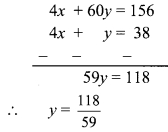∴ y = 2
Substituting y = 2 in equation (i),
x + 15y = 39
∴ x+ 15(2) = 39
∴ x + 30 = 39
∴ x = 39 – 30 = 9
∴ (9,2) is the solution of the given equations.

iii. $$\frac { 2 }{ x }$$ + $$\frac { 3 }{ y }$$ = 13
Multiplying both sides by 5,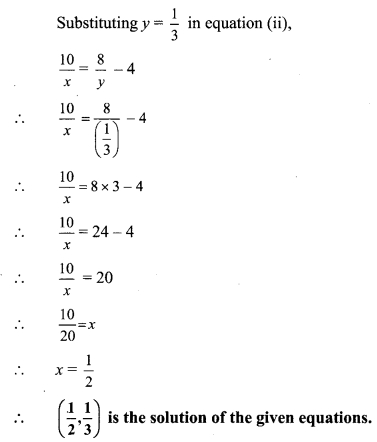Question 5.
A two digit number is 3 more than 4 times the sum of its digits. If 18 is added to this number, the sum is equal to the number obtained by interchanging the digits. Find the number.
Solution:
Let the digit in unit’s place be ‘x’ and the digit in ten’s place be ‘y’.According to the first condition,
a two digit number is 3 more than 4 times the sum of its digits.
10y + x = 4(x + y) + 3
∴ 10y + x = 4x + 4y + 3
∴ x – 4x + 10y – 4y = 3
∴ – 3x + 6y = 3
Dividing both sides by -3,
x – 2y = -1 …(i)
According to the second condition,
if 18 is added to the number, the sum is equal to the number obtained by interchanging the digits.
10y + x + 18= 10x + y
∴ x – 10x + 10y – y = -18
∴ – 9x + 9y = -18
Dividing both sides by – 9,
x – y = 2 ……(ii)
Subtracting equation (ii) from (i),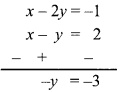∴ y = 3
Substituting y = 3 in equation (ii),
x – y = 2
∴ x – 3 = 2
∴ x = 2 + 3 = 5
∴ Original number = 10y + x
= 10(3) + 5
= 30 + 5
= 35
The required number is 35.

Question 6.
The total cost of 8 books and 5 pens is ₹ 420 and the total cost of 5 books and 8 pens is ₹321. Find the cost of 1 book and 2 pens.
Solution:
Let the cost of one book be ₹ x and the cost of one pen be ₹ y.
According to the first condition,
the total cost of 8 books and 5 pens is ₹ 420.
∴ 8x + 5y = 420 …(i)
According to the second condition, the total cost of 5 books and 8 pens is ₹ 321.
5x + 8y = 321 ….(ii)
Multiplying equation (i) by 5,
40x + 25y = 2100 …(iii)
Multiplying equation (ii) by 8,
40x + 64y = 2568 … (iv)
Subtracting equation (iii) from (iv),∴ y = 12
Substituting y = 12 in equation (i),
8x + 5y = 420
∴ 8x + 5(12) = 420
∴ 8x + 60 = 420
∴ 8x = 420 – 60
∴ 8x = 360
∴ x = $$\frac { 360 }{ 8 }$$
∴ x = 45
Cost of 1 book and 2 pens = x + 2y
= 45 + 2(12)
= 45 + 24
= ₹69
∴ The cost of 1 book and 2 pens is ₹69.

Question 7.
The ratio of incomes of two persons is 9 : 7. The ratio of their expenses is 4 : 3. Every person saves ₹ 200, find the income of each.
Solution:
Let the income of first person be ₹ x and that of second person be ₹ y.
According to the first condition,
the ratio of their incomes is 9 : 7.
∴ $$\frac { x }{ y }$$ = $$\frac { 9 }{ 7 }$$
∴ 7x = 9y
∴ 7x – 9y = 0 …….(i)
Each person saves ₹ 200.
Expenses of first person = Income – Saving = x – 200
Expenses of second person = y – 200
According to the second condition,
the ratio of their expenses is 4 : 3
∴ $$\frac { x – 200 }{ y – 200 }$$ = $$\frac { 4 }{ 3 }$$
∴ 3(x – 200) = 4(y – 200)
∴ 3x – 600 = 4y – 800
∴ 3x – 4y = – 800 + 600
∴ 3x – 4y = -200 …(ii)
Multiplying equation (i) by 4,
28x-36y =0 …(iii)
Multiplying equation (ii) by 9,
27x-36y = -1800 …(iv)
Subtracting equation (iv) from (iii),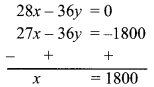Substituting x = 1800 in equation (i),
7x – 9y = 0
∴ 7(1800) – 9y = 0
∴ 9y = 7 x 1800
∴ y = $$\frac { 7 \times 1800 }{ 9 }$$
y = 7 x 200
∴ y = 1400
∴ The income of first person is ₹ 1800 and that of second person is ₹ 1400.

Question 8.
If the length of a rectangle is reduced by 5 units and its breadth is increased by 3 units, then the area of the rectangle is reduced by 9 square units. If length is reduced by 3 units and breadth is increased by 2 units, then the area of rectangle will increase by 67 square units. Then find the length and breadth of the rectangle.
Solution:
Let the length of the rectangle be ‘x’ units and the breadth of the rectangle be ‘y’ units.
Area of the rectangle = xy sq. units
length of the rectangle is reduced by 5 units
∴ length = x – 5
breadth of the rectangle is increased by 3 units
∴ breadth = y + 3
area of the rectangle is reduced by 9 square units
∴ area of the rectangle = xy – 9
According to the first condition,
(x – 5)(y + 3) = xy – 9
∴ xy + 3x – 5y – 15 = xy – 9
∴ 3x – 5y = -9 + 15
∴ 3x – 5y = 6 …(i)
length of the rectangle is reduced by 3 units
∴ length = x – 3
breadth of the rectangle is increased by 2 units
∴ breadth = y + 2
area of the rectangle is increased by 67 square units
∴ area of the rectangle = xy + 61
According to the second condition,
(x – 3)(y + 2) = xy + 67
∴ xy + 2x – 3y – 6 = xy + 67
∴ 2x – 3y = 67 + 6
∴ 2x – 3y = 73 …(ii)
Multiplying equation (i) by 3,
9x – 15y = 18 . ..(iii)
Multiplying equation (ii) by 5,
10x – 15y = 365 …(iv)
Subtracting equation (iii) from (iv), 10x- 15y= 365 9x-15y= 18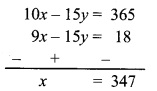Substituting x = 347 in equation (ii),
2x – 3y = 73
∴ 2(347) – 3y = 73
∴ 694 – 73 = 3y
∴ 621 = 3y
∴ y = $$\frac { 621 }{ 3 }$$
∴ y = 207
∴ The length and breadth of rectangle are 347 units and 207 units respectively.

Question 9.
The distance between two places A and B on a road is 70 kilometres. A car starts from A and the other from B. If they travel in the same direction, they will meet in 7 hours. If they travel towards each other they will meet in 1 hour, then find their speeds.
Solution:
Let the speed of the car starting from A (first car) be ‘x’ km/hr and that starting from B (second car) be ‘y’ km/hr. (x > y)
According to the first condition,
Distance covered by the first car in 7 hours = 7x km
Distance covered by the second car in 7 hours = 7y km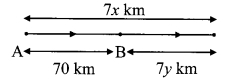If the cars are travelling in the same direction, 7x – 7y = 70
Dividing both sides by 7,
x – y = 10 …(i)
According to the second condition,
Distance covered by the first car in
1 hour = x km
Distance covered by the second car in 1 hour = y kmIf the cars are travelling in the opposite direction
x + y = 70 …(ii)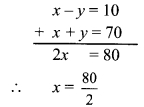∴ x = 40
Substituting x = 40 in equation (ii), x + y = 70
∴ 40 +y = 70
∴ y = 70 – 40 = 30
∴ The speed of the cars starting from places A and B are 40 km/hr and 30 km/hr respectively.

Question 10.
The sum of a two digit number and the number obtained by interchanging its digits is 99. Find the number.
Solution:
Let the digit in unit’s place be ‘x’ and the digit in ten’s place be ‘y’.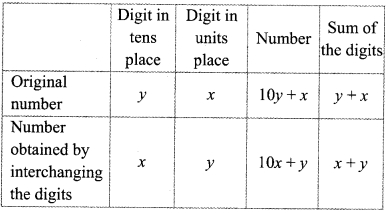According to the given condition,
the sum of a two digit number and the number
obtained by interchanging its digits is 99.
∴ 10y + x + 10x +y = 99
∴ 11x + 11y = 99
Dividing both sides by 11,
x + y = 9
If y = 1, then x = 8
If y = 2, then x = 7
If y = 3, then x = 6 and so on.
∴ The number can be 18, 27, 36, … etc.

Maharashtra Board Class 9 Maths Chapter 5 Linear Equations in Two Variables Practice Set 5 Intext Questions and Activities

Question 1.
On the glasses of following spectacles, write numbers such that (Textbook pg. no. 82)
i. Their sum is 42 and difference is 16.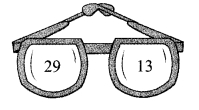ii. Their sum is 37 and difference is 11.iii. Their sum is 54 and difference is 20.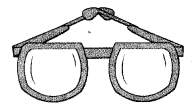iv. Their sum is … and difference is … .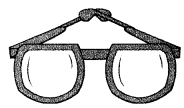ii. x + y = 37 and x – y = 11
∴ x = 24, y = 13
iii. x +y = 54 and x – y = 20
∴ x = 37, y =17

Question 2.
There are instructions written near the arrows in the following diagram. From this information form suitable equations and write in the boxes indicated by arrows. Select any two equations from these boxes and find their solutions. Also verify the solutions. By taking one pair of equations at a time, how many pairs can be formed ? Discuss the solutions for these pairs. (Textbook pg. no. 92)Here, if we take a pair of any two equations, we get following 6 pairs.

1. equation (i) and (ii)
2. equation (i) and (iii)
3. equation (i) and (iv)
4. equation (ii) and (iii)
5. equation (ii) and (iv)
6. equation (iii) and (iv)

Solution of each pair given above is (21, 15).
Here, all four equations are of same rectangle. By solving any two equations simultaneously, we get length and breadth of the rectangle.

Question 3.
Find the function.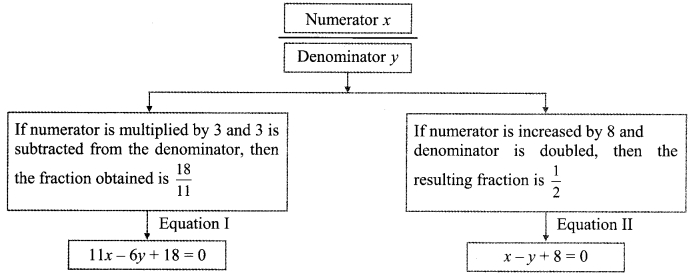∴ Given function = $$\frac { 6 }{ 14 }$$
Verify the answer obtained. (Textbook pg. no. 92)
For the fraction$$\frac { 6 }{ 14 }$$, if the numerator is multiplied by 3 and 3 is subtracted from the denominator, we get fraction $$\frac { 18 }{ 11 }$$.
Similarly, for the fraction $$\frac { 6 }{ 14 }$$, if the numerator is increased by 8 and the denominator is doubled, we get fraction $$\frac { 1 }{ 2 }$$.